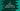# 3 different C programs to print odd numbers between 1 to 100## C program to print odd numbers between 1 to 100:

In this post, we will learn how to print all the odd numbers from 1 to 100 in different ways. A number is called an odd number if it is not divisible by 2. For example, 3, 5, 7, 9 etc. are odd numbers.

We will use one loop to iterate from 1 to 100. On each iteration of the loop the program will check if the number is an odd number or not and it will print it out if it is an odd number.

### How to use Modulo operator to check if a number is odd or not:

We will use the modulo operator to find if a number is an odd number or not. This operator, denoted by % is an arithmetic operator in C. We can use this operator to find out the remainder of a division operation.

For example, x%y will return the remainder of the x/y operation.

### Method 1: C to print all odd numbers in a range by using for loop:

Let’s try to use a for loop to find all odd numbers in between 1 to 100. The loop will start at 1 and end at 100. On each iteration, the program will check if the current value is an odd number or not. If it is odd, it will print its value. Else, it will start the next iteration.

Below is the complete program:

``````#include <stdio.h>

int main()
{
printf("Odd numbers between 1 to 100: ");

for (int i = 1; i <= 100; i++)
{
if (i % 2 != 0)
{
printf("%d ", i);
}
}
return 0;
}``````

The for loop in the program runs from i = 1 to i = 100. On each iteration, it checks if the current value of i is divisible by 2 or not i.e. if it is odd or not. If it is an odd value, it print its value.

If you run this program, it will print the below output:

``Odd numbers between 1 to 100: 1 3 5 7 9 11 13 15 17 19 21 23 25 27 29 31 33 35 37 39 41 43 45 47 49 51 53 55 57 59 61 63 65 67 69 71 73 75 77 79 81 83 85 87 89 91 93 95 97 99``

We can also add a simple logic to print the numbers separated by comma:

``````#include <stdio.h>

int main()
{
printf("Odd numbers between 1 to 100: ");

for (int i = 1; i <= 100; i++)
{
if (i % 2 != 0)
{
if(i != 1){
printf(", ");
}
printf("%d", i);
}
}
printf("\n");
return 0;
}``````

It will add a comma between each number and it will print the below output:

``Odd numbers between 1 to 100: 1, 3, 5, 7, 9, 11, 13, 15, 17, 19, 21, 23, 25, 27, 29, 31, 33, 35, 37, 39, 41, 43, 45, 47, 49, 51, 53, 55, 57, 59, 61, 63, 65, 67, 69, 71, 73, 75, 77, 79, 81, 83, 85, 87, 89, 91, 93, 95, 97, 99``

### Method 2: C to print all odd numbers in a range by using while loop:

We can replace the for loop of this program with a while loop. It will be similar to the above program, but the while loop will check the condition first and execute its body.

``````#include <stdio.h>

int main()
{
int i = 1;
printf("Odd numbers between 1 to 100: ");

while (i <= 100)
{
if (i % 2 != 0)
{
if (i != 1)
{
printf(", ");
}
printf("%d", i);
}
i++;
}
printf("\n");
return 0;
}``````

You will get the same output. We have initialized the value of i before the loop starts. At the end of each iteration, it increments the value of i by 1. The other parts of the program is exactly same as the previous example.

### Method 3: C to print all odd numbers in a range by using a for loop and with a different function:

Let’s use a different function to print the odd numbers. It will make the code clean and we can also call this function from different methods.

I will make this method to accept any n value as its parameter and print all odd numbers from 1 to n.

``````#include <stdio.h>

void printOdd(int n)
{
for (int i = 1; i <= n; i++)
{
if (i % 2 != 0)
{
if (i != 1)
{
printf(", ");
}
printf("%d", i);
}
}
printf("\n");
}
int main()
{
int i = 1;
printf("Odd numbers between 1 to 100: ");
printOdd(100);
return 0;
}``````

Here,

• The printOdd function takes one parameter, the value of n and it prints the odd numbers from 1 to n.
• For this example, we are printing the odd numbers from 1 to 100. So, we called this method with 100 as the parameter.

Output:

``Odd numbers between 1 to 100: 1, 3, 5, 7, 9, 11, 13, 15, 17, 19, 21, 23, 25, 27, 29, 31, 33, 35, 37, 39, 41, 43, 45, 47, 49, 51, 53, 55, 57, 59, 61, 63, 65, 67, 69, 71, 73, 75, 77, 79, 81, 83, 85, 87, 89, 91, 93, 95, 97, 99``

You can pass any value of n to find all odd numbers between 1 to n.